# Home Work Set # 9, Physics 217, Due: November 14, 2001

### Problem 1

An electric dipole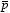, pointing in the y direction, is placed midway between two large conducting plates, as shown in Figure 1. Each plate makes a small angle θ with respect to the x axis, and they are maintained at potentials ±V. What is the direction of the net force on? Explain!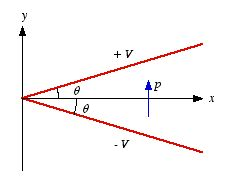Figure 1. Problem 1.

### Problem 2

A point charge q is embedded at the center of a sphere of linear dielectric material (with susceptibility χe and radius R).
a) Find the electric field, the polarization and the bound charge.
b) What is the total bound charge on the surface?
c) Where is the compensating negative bound charge located?

### Problem 3

At the interface between one linear dielectric and another linear dielectric the electric field lines bend (see Figure 2). Show that tanθ2/tanθ1 = ε2/ε1, assuming that there is no free charge at the boundary.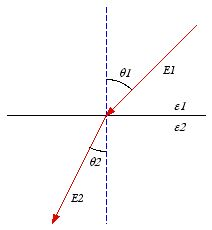Figure 2. Problem 3.

### Problem 4

a) Find the force on a square loop placed as shown in Figure 3a, near an infinite straight wire. Both the loop and the wire carry a steady current I.
b) Find the force on a triangular loop placed as shown in Figure 3b, near an infinite straight wire. Both the loop and the wire carry a steady current I.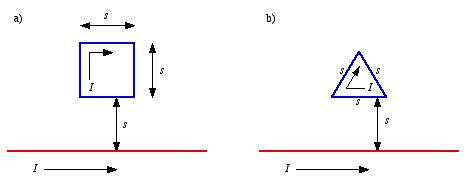Figure 3. Problem 4.

### Problem 5

Suppose the magnetic field in some region has the form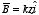where k is some constant. Find the force on a square loop of side s, lying in the yz plane, centered at the origin, which carries a current I.